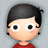#### 学习笔记TF004:张量表示、类型、形状、计算

``````import tensorflow as tf
a = tf.constant([[[1,2,3],[4,5,6]],[[7,8,9],[1,2,3]]], name="input_a")
b = tf.reduce_prod(a, name="prod_b")#定义节点b，创建归约乘积Op,接收一个N维张量输入,输出张量所有分量(元素)的乘积,以prod_b标识。
c = tf.reduce_sum(a, name="sum_c")#定义节点c，创建归约求和Op,接接收一个N维张量输入,输出张量所有分量(元素)的求和,以sum_c标识。
sess = tf.Session()
out = sess.run(d)
writer = tf.summary.FileWriter('./my_graph', sess.graph)
writer.close()
sess.close()
``````

TensorFlow可接收Python数值、布尔值、字符串、数组。单个数值转化为0阶张量（标量）。数值列表转化为1阶张量（向量）。由列表构成的列表转化为2阶张量（矩阵）。 TensorFlow数据类型：float32､float64､int8､int16､int32､int64､uint8､string､bool､cpmples64(实部32位虚部32位的64位复数)､qint8(有符号用于量化Op)､qint32､quint8(无符号用于量化Op)。

NumPy专为操作N维数组设计的科学计算软件包，是数据科学通用语言。TensorFlow数据类型基于NumPy。任何NumPy数组都可以传递给TensorFlow Op。指定所需数据类型代价最小。NumPy没有与tf.string字符串精确对应的数据类型。TensorFlow可以导入NumPy的字符串数组，但不能在NumPy中显式指定dtype属性。Session.run()方法返回的张量都是NumPy数组。不要用TensorFlow的数据类型初始化NumPy数组。推荐用NumPy手工指定Tensor对象。 张量Shape属性，表示张量的维(阶)数和每一维的长度。张量形状是包含有序整数集的列表(list)或元组(tuple)。元素数量即维数，元素数值即每一维的长度。.shape()创建获取张量形状Op。

``````import tensorflow as tf
#指定0阶张量(标量)形状
s_0 = 1
#指定1阶张量(向量)形状
s_1_list = [1,2,3]
s_1_tuple = (1,2,3)
#指定2阶张量(矩阵)形状
s_2 = [[2,3],[2,3]]
#指定任意维数任意长度的张量形状
s_any = None
shape = tf.shape(s_2, name="mystery_shape")#创建获取张量形状Op,接收一个张量，输出张量形状,以mystery_shape标识。
sess = tf.Session()
sess.run(shape)
``````

Operation，Op，Tensor对象运算节点，返回张量。在Python中调用创建Op的构造方法，传入所需所有Tensor输入，以及正确创建Op所需属性，返回创建Op的输出(张量)句柄。name属性用描述性字符串指代Op。